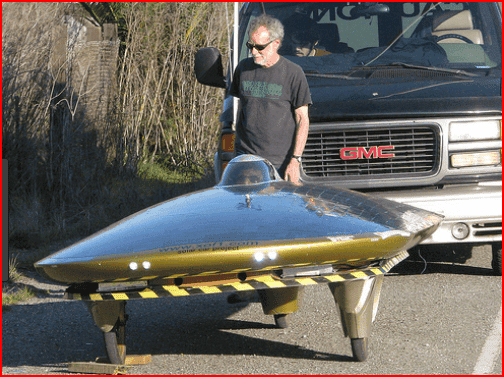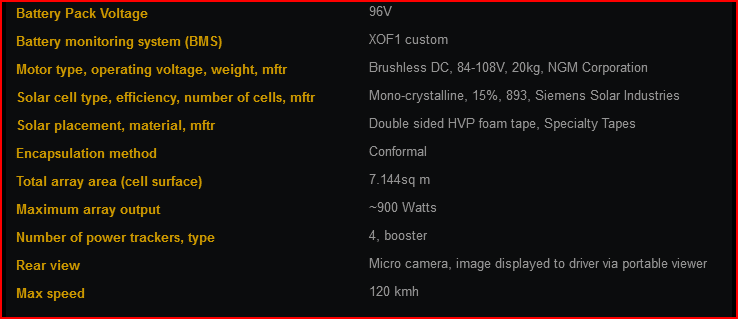# How can I calculate the maximum speed of a go kart?

• Xander
One way to measure the power required is to tow the cart with a long tow rope. Measure the tension in the tow rope at various velocities. Then...This reply is so simplistic that it implies you have not really processed what you have been told. So I'll be succinct and perhaps a bit provocative:Gear ratio does not affect top speed, if you properly approach the design. Based on what you know about power and what you were told above, do you know why?f

#### Xander

Hello, I'm going to build a solar-powered go-kart. To determine the sprocket that I want to use I need a formula to calculate the top speed which also considers vehicle weight and other resistance forces. All the formulas I found just used sprocket tooth and rpm to calculate top speed but of course the weight of the kart and other factors also influence the top speed. Does anyone have a formula with which I can calculate this?

Hello, I'm going to build a solar-powered go-kart. To determine the sprocket that I want to use I need a formula to calculate the top speed which also considers vehicle weight and other resistance forces. All the formulas I found just used sprocket tooth and rpm to calculate top speed but of course the weight of the kart and other factors also influence the top speed. Does anyone have a formula with which I can calculate this?
It's really difficult to calculate this from scratch. It depends on wind resistance rolling resistance and drivetrain friction. I suggest looking at the specs of commercially available gokarts and baking it off that.

Two things can limit the maximum speed of a wheel-powered vehicle: Power or traction force.

The principal resistance than the engine has to fight is the aerodynamic drag (##F_d = \frac{1}{2}\rho C_dAv^2##). The power required to fight that force is then ##P = F_d \times v##. So, assuming the engine is at its maximum power ##P_{max}##:
$$v_{max} = \sqrt{\frac{P_{max}}{\frac{1}{2}\rho C_dA}}$$
But no matter how much power you have, the wheels must be able to transmit the force to the ground (i.e. the wheels must not spin). So the drag force cannot be higher than the friction force available at the wheels (##\mu N_r##, where ##N_r## is the normal force acting on the rear wheels, for the case of a rear wheel drive). Based on this, the maximum speed is:
$$v_{max} = \sqrt{\frac{\mu N_r}{\frac{1}{2}\rho C_dA}}$$
And ##N_r## can be found with (considering weight transfer):
$$N_r = \frac{W_r/W}{1- \mu\frac{h}{L}}mg$$
Where ##W_r/W## is the portion of the vehicle's weight resting on the rear wheels, ##h## is the height of the center of gravity, and ##L## is the vehicle's wheelbase.

For more details - and more complete equations - see this page and this one.

•Tom.G
Gear ratio also has effect on the top speed how can I calculate that?

If the system is "rev limited" then the max speed in meters per second is..

Motor rpm * gear ratio * wheel circumference in meters / 60

However that assumes the motor is capable of delivering the power required to go that fast.

If the system is power limited then you need to know the power required. One way to measure the power required is to tow the cart with a long tow rope. Measure the tension in the tow rope at various velocities. Then..

Power in Watts = tension in Newtons * velocity in meters per second

Plot a graph of power Vs velocity.

Your motor and gearing needs to be capable of producing more power than that at all velocities (eg a higher curve). The excess power is needed to accelerate. Where the available power curve crosses the required power curve is the maximum possible velocity.

•jim hardy
Gear ratio also has effect on the top speed how can I calculate that?
This reply is so simplistic that it implies you have not really processed what you have been told. So I'll be succinct and perhaps a bit provocative:

Gear ratio does not affect top speed, if you properly approach the design. Based on what you know about power and what you were told above, do you know why?

I think it's also worth doing a sanity check on the whole concept of a solar powered go-kart. How big is the kart? How much power could you generate if the whole thing was covered in solar panels?

•russ_watters
I think it's also worth doing a sanity check on the whole concept of a solar powered go-kart. How big is the kart? How much power could you generate if the whole thing was covered in solar panels?
@Xander -- have you read through pages like this?

https://www.xof1.com/specifications.php#### Attachments

Yeah, but that's also 5m long and 1.8m wide, and clearly designed for aerodynamics and low rolling resistance. 900 watts is still only around 1 horsepower, which is really not very much for a human-carrying vehicle. The acceleration will be sedate at best.

•russ_watters
Agreed, I mostly just wanted to get the OP thinking along the lines of what his kart specifications are, so he can get closer to calculating the details like power, gearing, aero, etc.•russ_watters and CWatters
If the system is "rev limited"
Assuming a typical DC motor, it's unlikely to be rev limited, as peak torque occurs at 0 rpm, and linearly decreases to zero at maximum no load rpm. Peak power occurs at about 1/2 the maximum no load rpm.

If the system is power limited then you need to know the power required. One way to measure the power required is to tow the cart with a long tow rope. Measure the tension in the tow rope at various velocities.
It might be easier to convert motor torque into force at the contact patches of the driven tires (after drive train losses), and just compare force from motor, versus force measured from the tow rope, at various velocities.

Unless solar panels have improved much from the vehicles like the one linked to above:

https://www.xof1.com/specifications.php

the top speed will fairly low unless the design mimics that vehicle. 900 watts is about 1.2 hp, and that is a fairly large solar panel on that vehicle. I'm also wondering what the limits are in terms of cross winds before it becomes dangerous for that vehicle.

•berkeman, CWatters and russ_watters
The motor I’ll he using is a 250watt 24v motor. The rpm is 2650.

I know that the top speed won’t be high, around 5 mph would be nice already

The motor I’ll he using is a 250watt 24v motor. The rpm is 2650.

that is a 550-watt panel

Why not use a 550 watt capable motor if you have 550 watts potentially available?

because the panel never generates 550 watt.

I know that the top speed won’t be high, around 5 mph would be nice already

This seems pretty pessimistic. Suppose you have a 80% efficiency electric motor (it should be at least that high), so you have 200 W to play with. At this speed you can really ignore the air friction (The power needed is proportional to the third power of the speed). All the power must go into rolling resistance. The power needed is F * v. (friction force times speed). Since the speed is only 2.24 m/s, the friction force must be 90N. Suppose the thing weighs 200 kg, including driver, The normal force is 9.8 * 200 is 1960N. The rolling resistance coefficient, is 0.046, which is about 3 times the worst value I can find for car wheels. Your go-kart wouldn't accelerate on a 4.6% downslope. Do you drive in sand?

So how fast do you think it'll go?

#### Attachments

•jack action